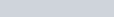The bisection method is a simple extension to the change of sign method where an interval in which a root lies is continually bisected (cut in half) until the root is found to the required degree of accuracy.

The following example is used to demonstrate this:

### Example:

1. Find the root ofto 1 decimal place using the bisection method, given that the root lies between 0 and 1.
The bisection method is a simple extension to the change of sign method where an interval in which a root lies is continually bisected (cut in half) until the root is found to the required degree of accuracy. The following example is used to demonstrate this: Example: Find the root of [latex]x^3+2x-